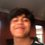# MITPrimes 2018 Question 6

Let $P$ be a polynomial with integer coefficients and at least $3$ simple roots. Is it true that $P(n)$ is powerful only finitely often?

Can you guys tell me how you would approach this problem?

Source: MITPrimes 2018Note by Vishruth Bharath
3 years, 5 months ago

This discussion board is a place to discuss our Daily Challenges and the math and science related to those challenges. Explanations are more than just a solution — they should explain the steps and thinking strategies that you used to obtain the solution. Comments should further the discussion of math and science.

When posting on Brilliant:

• Use the emojis to react to an explanation, whether you're congratulating a job well done , or just really confused .
• Ask specific questions about the challenge or the steps in somebody's explanation. Well-posed questions can add a lot to the discussion, but posting "I don't understand!" doesn't help anyone.
• Try to contribute something new to the discussion, whether it is an extension, generalization or other idea related to the challenge.

MarkdownAppears as
*italics* or _italics_ italics
**bold** or __bold__ bold
- bulleted- list
• bulleted
• list
1. numbered2. list
1. numbered
2. list
Note: you must add a full line of space before and after lists for them to show up correctly
paragraph 1paragraph 2

paragraph 1

paragraph 2

[example link](https://brilliant.org)example link
> This is a quote
This is a quote
    # I indented these lines
# 4 spaces, and now they show
# up as a code block.

print "hello world"
# I indented these lines
# 4 spaces, and now they show
# up as a code block.

print "hello world"
MathAppears as
Remember to wrap math in $$ ... $$ or $ ... $ to ensure proper formatting.
2 \times 3 $2 \times 3$
2^{34} $2^{34}$
a_{i-1} $a_{i-1}$
\frac{2}{3} $\frac{2}{3}$
\sqrt{2} $\sqrt{2}$
\sum_{i=1}^3 $\sum_{i=1}^3$
\sin \theta $\sin \theta$
\boxed{123} $\boxed{123}$

Sort by:

After doing some research, I've found what a "powerful" number is. Basically, if we let a number be represented by $m$ such that if $p|m$, then $p^2|m$ is called a "powerful" number. The first few powerful numbers are $1,4,8,9,16,25,27,32, 36, \dots$

Powerful numbers are always in the form of $a^2b^3$ for $a,b \geq 1$.

- 3 years, 5 months ago

It's about the $\text{abc}$ conjecture for powerful numbers.

- 3 years, 5 months ago

Ok!! That's some new information....Thanks......Dude where did you find all this??

- 3 years, 5 months ago

@Aaghaz Mahajan I found it on WolfRam

- 3 years, 5 months ago

What do you mean by "Powerful" ?? Also, what are "simple roots" ?? Are they integral roots??

- 3 years, 5 months ago

Same, I have doubts about what makes something "powerful." Also, I believe simple roots are not integral roots. @Chew-Seong Cheong what do you think?

- 3 years, 5 months ago

Well, maybe the question paper had some previously stated criteria for defining these terms........I even checked on the net and couldn't find an aswer to this query.......

- 3 years, 5 months ago